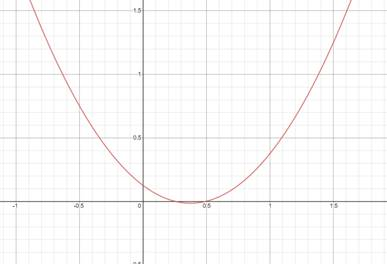# Solve the equation graphically in the given interval.### Precalculus: Mathematics for Calcu...

6th Edition
Stewart + 5 others
Publisher: Cengage Learning
ISBN: 9780840068071### Precalculus: Mathematics for Calcu...

6th Edition
Stewart + 5 others
Publisher: Cengage Learning
ISBN: 9780840068071

#### Solutions

Chapter 1.9, Problem 45E
To determine

## Solve the equation graphically in the given interval.

Expert Solutionx=1,2,3

### Explanation of Solution

Given information:

x36x2+11x4=0

[1,4]

Formula used:

Plot the graph and approximate the solution of x.

Graphically:

Making Graph of x36x2+11x4=0 and finding approximate value of x.Graph intersect x-axis at (1,0),(2,0),(3,0) in the interval of [1,4]

x=1,2,3

### Have a homework question?

Subscribe to bartleby learn! Ask subject matter experts 30 homework questions each month. Plus, you’ll have access to millions of step-by-step textbook answers!